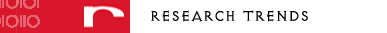Author ResourcesAuthor GatewayArticle submission guidelines

 Editor ResourcesEditor/Referee Gateway

 Agents/DistributorsRegional Subscription Agents/DistributorsCurrent Topics in Electrochemistry  → Volumes  →  Volume 4 Abstract The Phasance concept: a review Jean Jacquelin Pages: 127 - 136 Number of pages: 10 Current Topics in ElectrochemistryVolume 4  Copyright © 1997 Research Trends. All rights reserved ABSTRACT The Phasance concept is introduced using either a practical or a theoretical approach. The potential difference, function of time, V (t), causing a current, I(t), to flow in a circuit element, can be expressed in terms of the fractional derivative of the current, thanks to the Rieman-Liouville transform: V = Pφ.dvI/dtv, where Pφ is the Phasance and v is the degree of fractional derivation. In the sinusoidal case, the relationship between the impedance (Z) and the Phasance is Z = Pφ(iω)v, where v is related to the phase angle displacement (φ). A basic element, so-called Phasor (Pφ,v=2φ/π), is described in the case of constant Pφ and v. The key characteristic of this element is a constant energy efficiency. The consideration of Resistance, Capacitance, Inductance as boundary cases of Phasance, leads to a more general approach in mathematical treatments. Theoretical and practical examples are presented, which result from a review of information, notions, and ideas derived from the literature. The unified concept of Phasance is especially useful in Electrochemistry. Thanks to this theoretical basis, one can understand why some kinds of complex impedance behaviours are so common and are likely to be caused by many different phenomena. This is also the starting point for the derivation of a number of models and equivalent circuits.

 search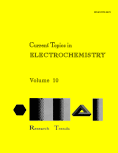E-CommerceBuy this articleBuy this volumeSubscribe to this title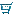Shopping Cart

 Quick LinksLogin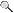Search Products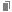Browse in Alphabetical Order : JournalsSeries/BooksBrowse by Subject Classification : JournalsSeries/Books

 Miscellaneous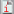Ordering InformationDownloadable Forms